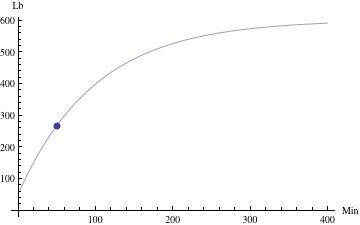# Initially 50 pounds of salts is dissolved in a large tank having 300 gallons of water. A brine solution is pumped into the tank at a rate of 3 gal/min and a well-stirred solution is then pumped out at the same rate?

Suppose the total amount of salts in the tank is s[t] pounds, where t is time in minutes. We are told that
s = 50 pounds
The rate of increase of s[t] is
(3 gal/min)*(2 lb/gal) = 6 lb/min
The rate of decrease of s[t] (in pounds per minute) is
s/(300 gal)*(3 gal/min)
Expressing these relationships using a differential equation, we have
s'[t] = 6 - (1/100)*s,
s = 50
We recognize that the solution to this linear non-homogeneous equation with constant coefficients will be of the form
s[t] = c1e^(a*t) + c0  for some values of a and constants cn.
Substituting this into the differential equation above, and matching coefficients, we get
c1*a*e^(a*t) = 6 - (1/100)*c1*e^(a^t) - (1/100)*c0   c1*a = -(1/100)*c1    (match coefficients of e^(a*t))
a = -1/100   0 = 6 - (1/100)*c0    (match constants)
c0 = 600   c1*e^(-0/100) + 600 = 50    (evaluate for initial condition)
c1 = 50 - 600 = -550 The salt quantity is
s[t] = 600 - 550e^(-t/100)) After 50 minutes, the salt quantity in the tank will be
s = 600 - 550*e^(-1/2)
s ≈ 266.4 pounds of salts The quantity present as t gets very large will be
s[infinity] = 600 poundsthanked the writer.